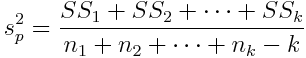The pooled variance is the average of several group variances, in which each group variance is weighted for the size of the group.Notice that the calculation of the pooled variance is analagous to the calculation of the "pooled" or weighted mean, since "ess-ess" is equal to "en times the variance." Here we divide the summed sums of squares by degrees of freedom rather than the number of subjects, however. For this calculation, degrees of freedom is equal to the total number of subjects minus the number of groups, a good rule to commit to memory for calculating pooled variances. Return To Main Page